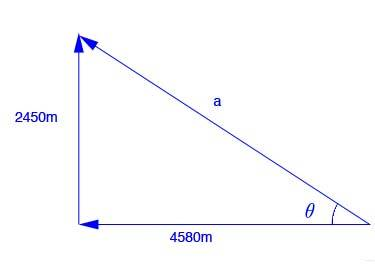# Can you explain the Pythagorean theorem in two and three dimensions step by step?

The same concept applies to 2D and 3D.

We first learned about the Pythagorean Theorem when trying to find the lengths of sides in two dimensional objects, like so:You would just use the standard formula to find what a is. And in this picture, since a is the hypotenuse, then the formula will be rewritten as a^2 = b^2 + c^2.

Pythagorean Theorem use for 3-Dimensional objects would mean to apply the same formula, more than once, to different parts of the object  to find more than one unknown length. This can get a bit tedious if you use it to find the length of a diagonal, if the shape is a rectangle or square or whatever. But as long as you understand the theorem and which variable represents the hypotenuse and which ones represent the side lengths, you'll be able to figure it out.

thanked the writer.